# How to Sum if Equal to Many Items or A Range in Excel

If we want to sum numbers from a range with criteria, we often select SUMIF of SUMIFS functions as the first choice to create a formula. The criteria can be a number or an array or a collection of some items. In this article, we will show you to sum numbers based on a range of items. In fact, there are many functions can be applied to help us to figure out this problem, and in real life based on different situations, you can select different functions. But in this article, we will show you the way to resolve this problem by using SUMIF/SUMPRODUCT functions combination. We will introduce you the syntax, arguments and basic usage of these functions, and let you know the working process of our formula.

## EXAMPLE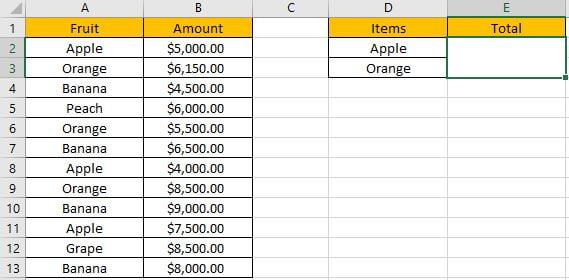Refer to the left-hand side table, we can see some kinds of fruits are listed in “Fruit” column. And amounts are listed in “Amount” column. In the right-hand side table, we list the items we want to sum amounts for them. In previous instance, we have learned how to sum amounts for one item only, for example, we can apply SUMIF of SUMIFS function to create a formula “=SUMIF(A2:A13,D2,B2:B13)” to sum amounts for “Apple”.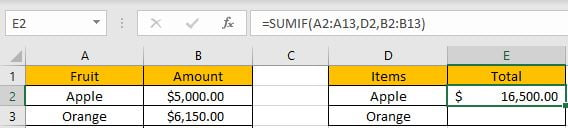But in this case, sum criteria contain two items “Apple” and “Orange”, and the total amount is recorded in one cell and it covers the two items. Thus, only applying SUMIF function cannot figure out our problem, even we can create a formula like “=SUMIF(A2:A13,D2,B2:B13)+SUMIF(A2:A13,D3,B2:B13)” to calculate total amount, it is clear but complex, and when there are multiple items, the formula will be very long. So, we need to find a proper way to sum. In fact, there are many ways to sum numbers based on criteria contain a range reference, for this instance, we will show you to sum amounts for many items by SUMPRODUCT and SUMIF functions.

Before designing a formula, we can name range references.

Select range A2:A13, in name box enter “Fruit”, then press Enter.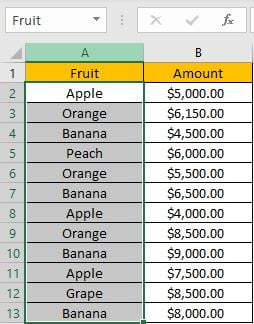Select range B2:B13, in name box enter “Amount”, then press Enter.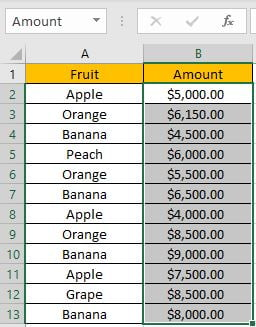Select range D2:D3, in name box enter “Items”, then press Enter.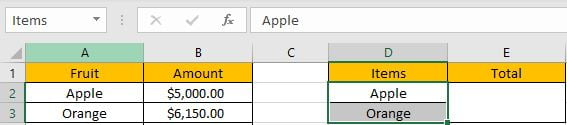## CREATE A FORMULA with SUMPRODUCT & SUMIF FUNCTIONS

### 1.STEPS

Step 1: In E2, enter the =SUMPRODUCT(SUMIF(Fruit,Items,Amount)).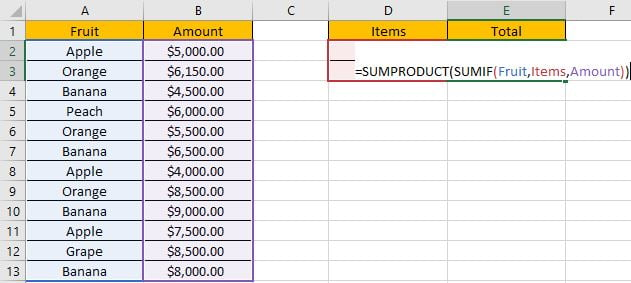You can enter named range like “Fruit” into your formula directly, or you can also select the range by dragging the handle as well.

Step 2: Press Enter after typing the formula.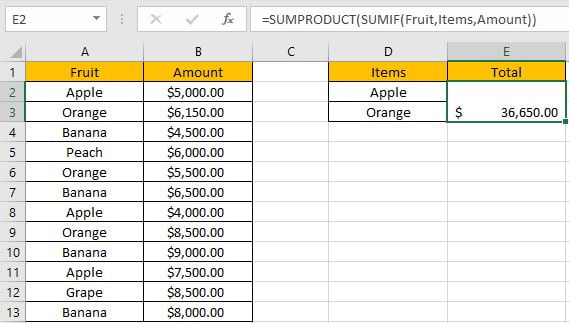We can see in column A, cell A2, A3, A6, A8, A9 and A11 meet our criteria, so we just need to sum numbers from B2, B3, B6, B8, B9 and B11, so the total amount is 5000+6150+5500+4000+8500+7500=36650. The formula works correctly.

### 2.FUNCTION INTRODUCTION

SUMIF function can be seen as SUM+IF. It can sum up numbers by specific condition.

Syntax:

` =SUMIF(criteria_range, criteria, sum_range)`

SUMPRODUCT function can return sum of products.

Syntax:

` =SUMPRODUCT(array1, [array2], [array3], ...)`

For both SUMPRODUCT and SUMIF functions, they support wildcards like asterisk ‘*’ and question mark ‘?’, also support logical operators like ‘>’,’<’. If wildcards or logical operators are required, they should be enclosed into double quotes (““).

### 3.ALL ARGUMENTS

In this case, SUMIF is included in SUMPRODUCT formula as its argument.

SUMIFS – RANGE

Range reference “Fruit” is the criteria range.

In the formula bar, select “Fruit”, press F9, values in this range are expanded in an array.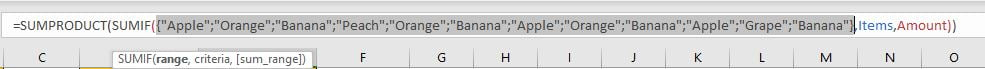SUMIFS – CRITERIA

As we want to sum amount for “Apple” and “Orange” together, so the criteria here is a range reference “Items”.

In the formula bar, select “Items”, press F9, items in this range are expanded in an array.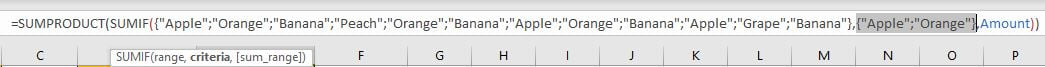As we said we can use “=SUMIF(A2:A13,D2,B2:B13)+SUMIF(A2:A13,D3,B2:B13)” to sum total for both two items, in fact we can use an array constant like {D2;D3} to shorten the formula.

Note: an array constant can provide a set of values, and the values are hard-coded. It is frequently used in array formula.

SUMIF – SUM RANGE

Range reference “Amount” is the “sum range”.

In the formula bar, select ‘Amount’, press F9, amounts in this range are expanded in an array.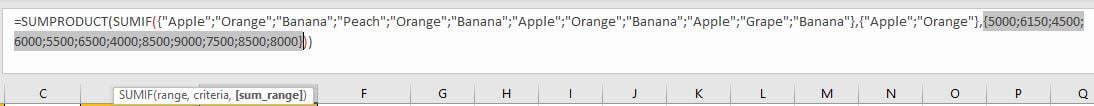### 4.HOW THE FORMULA WORKS

After explaining each argument in the formula, now we will show you how the formula works with these arguments.

After expanding values in each range reference, in the formula bar, the formula is displayed as:

`=SUMPRODUCT(SUMIF({"Apple";"Orange";"Banana";"Peach";"Orange";"Banana";"Apple";"Orange";"Banana";"Apple";"Grape";"Banana"},{"Apple";"Orange"},{5000;6150;4500;6000;5500;6500;4000;8500;9000;7500;8500;8000}))`

A pair of criteria range and criteria:

`{"Apple";"Orange";"Banana";"Peach";"Orange";"Banana";"Apple";"Orange";"Banana";"Apple";"Grape";"Banana"}`

– Criteria Range

{“Apple”;”Orange”} – Criteria (more than one items)

If items from criteria range match either of criteria “Items”, “True” will be recorded in the array, otherwise, “False” will be recorded. So, after comparing with “Apple” and “Orange” separately, the original criteria range is split to two new arrays which only contains “True” and “False”.

{True;False;False;False;False;False;True;False;False;True;False;False} – compare with “Apple”

{False;True;False;False;True;False;False;True;False;False;False;False} – compare with “Orange”

For the following logical operation, “True” is coerced to “1” and “False” is coerced to “0”. So, values in the array are converted to numbers:

{1;0;0;0;0;0;1;0;0;1;0;0} – compare with “Apple”

{0;1;0;0;1;0;0;1;0;0;0;0} – compare with “Orange”

Sum amount of “Apple” refer to sum range:

{1;0;0;0;0;0;1;0;0;1;0;0} – filter “Apple” in criteria range

{5000;6150;4500;6000;5500;6500;4000;8500;9000;7500;8500;8000} – sum_range

Multiply the values from the two arrays in the same position. Then we save the products in an array:

{5000;0;0;0;0;0;4000;0;0;7500;0;0}

Sum amount of “Orange” refer to sum range:

{0;1;0;0;1;0;0;1;0;0;0;0} – filter “Orange” in criteria range

{5000;6150;4500;6000;5500;6500;4000;8500;9000;7500;8500;8000} – sum_range

Multiply the values from the two arrays in the same position. Then we save the products in an array:

{0;6150;0;0;5500;0;0;8500;0;0;0;0}

In this case if we directly select SUMIF part and press F9, array {16500;20150} is returned.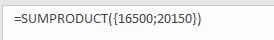We can also use SUM function to replace SUMPRODUCT function in this case.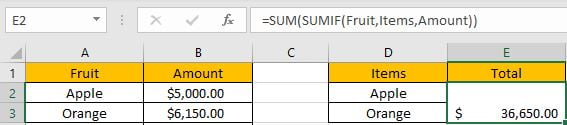## SUMMARY

1. SUMIF function can handle only one pair of criteria range and criteria. Sum range is the last argument among all arguments.

2. SUMPRODUCT function can return the sum of products.

3. They all support wildcards, logical operators.

### Related Functions

• Excel SUMPRODUCT function
The Excel SUMPRODUCT function multiplies corresponding components in the given one or more arrays or ranges, and returns the sum of those products.The syntax of the SUMPRODUCT function is as below:= SUMPRODUCT (array1,[array2],…)…
• Excel SUMIFS Function
The Excel SUMIFS function sum the numbers in the range of cells that meet a single or multiple criteria that you specify. The syntax of the SUMIFS function is as below:=SUMIFS (sum_range, criteria_range1, criteria1, [criteria_range2, criteria2], …)…
• Excel SUMIF Function
The Excel SUMIF function sum the numbers in the range of cells that meet a single criteria that you specify. The syntax of the SUMIF function is as below:=SUMIF (range, criteria, [sum_range])…